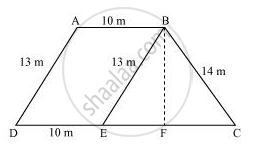# A field is in the shape of a trapezium whose parallel sides are 25 m and 10 m. The non-parallel sides are 14 m and 13 m. Find the area of the field. - Mathematics

A field is in the shape of a trapezium whose parallel sides are 25 m and 10 m. The non-parallel sides are 14 m and 13 m. Find the area of the field.

#### SolutionDraw a line BE parallel to AD and draw a perpendicular BF on CD.

It can be observed that ABED is a parallelogram.

BE = AD = 13 m

ED = AB = 10 m

EC = 25 − ED = 15 m

For ΔBEC,

Semi-perimeter,

s=(13+14+15)/2=21 m

By Heron’s formula,

"Area of triangle "=sqrt(s(s-a)(s-b)(s-c))

"Area of ΔBEC "=[sqrt(21(21-13)(21-14)(21-15))]m^2

=[sqrt(21(8)(7)(6))]m^2

= 84 m2

"Area of ΔBEC "=1/2xxCExxBF

rArr84=1/2xx15xxBF

rArrBF=168/15=11.2 m

Area of ABED = BF × DE = 11.2 × 10 = 112 m2

Area of the field = 84 + 112 = 196 m2

Concept: Application of Heron’s Formula in Finding Areas of Quadrilaterals
Is there an error in this question or solution?
Chapter 12: Heron's Formula - Exercise 12.2 [Page 207]

#### APPEARS IN

NCERT Class 9 Maths
Chapter 12 Heron's Formula
Exercise 12.2 | Q 9 | Page 207

Share